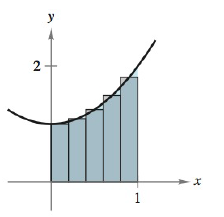Chapter 5, Problem 104RE### Calculus: An Applied Approach (Min...

10th Edition
Ron Larson
ISBN: 9781305860919

#### Solutions

Chapter
Section### Calculus: An Applied Approach (Min...

10th Edition
Ron Larson
ISBN: 9781305860919
Textbook Problem
3 views

# Approximating the Area of a Plane Region In Exercises 103 and 104, use the rectangles to approximate the area of the region. Compare your result with the exact area obtained using a definite integral. f ( x ) = x 2 + 1 ,     [ 0 , 1 ]To determine

To calculate: The area of the region bounded by the graph of the function f(x)=x2+1 in the interval [0,1] with the help of midpoint rule for rectangle to approximation and compare the result with exact area.

Explanation

Given information:

The functions is f(x)=x2+1 and the provided intervals is [0,1]. The provided figure is shown below,

Formula used:

Steps to solve a definite integral abf(x)dx with the help of midpoint rule.

Step 1: For a given interval [a,b] divide it into n subintervals with having width of,

Δx=ban

Step 2: Evaluate the midpoint for the given subinterval. Midpoints={x1,x2,x3,xn}

Step 3: Find the value of f at each midpoint and make the sum as shown below:

abf(x)dxban[f(x1)+f(x2)+f(x3)++f(xn)]

Fundamental theorem of calculus,

abf(x)dx=F(x)]ab=F(b)F(a)

Calculation:

Consider the provided function,

f(x)=x2+1

The graph is divided into 5 rectangles from 0 to 1 therefore. The intervals are [0,1] with n=5

Now divide the provided interval into 5 subparts as shown below,

Δx=105=15

Therefore the 5 subintervals are, [0,15],[15,25],[25,35],[35,45] and [45,1].

Now find the mid points of these intervals because each subinterval has a width of 15. Therefore, the mid points of these intervals are 110,310,12,710 and 910.

Here the mid points 110,310,12,710 and 910 lies in the middle of [0,15],[15,25],[25,35],[35,45] and [45,1] intervals respectively

### Still sussing out bartleby?

Check out a sample textbook solution.

See a sample solution

#### The Solution to Your Study Problems

Bartleby provides explanations to thousands of textbook problems written by our experts, many with advanced degrees!

Get Started

#### Calculate y'. 47. y = cosh1(sinh x)

Single Variable Calculus: Early Transcendentals, Volume I

#### In problems 63-73, factor each expression completely. 70.

Mathematical Applications for the Management, Life, and Social Sciences

#### The polar coordinates of the point P in the figure at the right are:

Study Guide for Stewart's Single Variable Calculus: Early Transcendentals, 8th